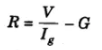# The conversion of a moving coil galvanometer into a voltmeter is done by (1) introducing a resistance of large value in series (2) introducing a resistance of small value in parallel (3) introducing a resistance of large value in parallel (4) introducing a resistance of small value in series

The conversion of a moving coil galvanometer into a voltmeter is done by (1) introducing a resistance of large value in series (2) introducing a resistance of small value in parallel (3) introducing a resistance of large value in parallel (4) introducing a resistance of small value in series

Answer: (1) introducing a resistance of large value in series

A galvanometer is an ideal device that is capable of detecting even the weakest electric currents in an electric circuit. It features a coil suspended or pivoted between concave pole faces of a strong laminated horseshoe magnet.

• The galvanometer shows the deflection when an electric current is passed through the coil.
• The deflection is directly proportional to the current passed.
• A voltmeter is an instrument used for estimating the electrical potential difference between 2 points in an electric circuit.
• A very high resistance known as series resistance is placed in series with the galvanometer to convert it into a voltmeter.

### Series resistance required for conversion of galvanometer into voltmeter(0)(0)